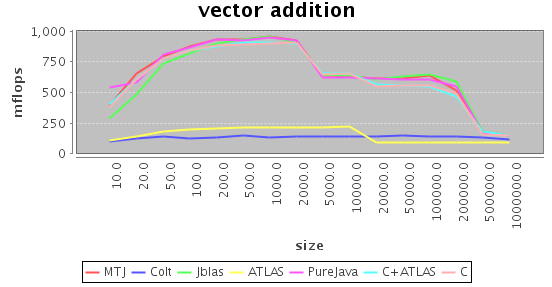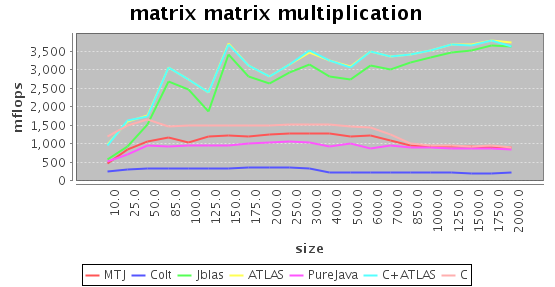# Some Benchmark Numbers for jblas

Monday, April 06, 2009

The last few days I’ve been putting together a benchmarking tool for jblas which I’ll hope to release to the public as soon as I’ve figured out how to best package that beast.

It compares my own matrix library, jblas against the Matrix Toolbox for Java, Colt, as well as simple implementations in C and Java, and, of course, ATLAS.

All experiments were run on my Laptop which sports an Intel Core 2 CPU (T7200) with 2GHz. Maybe the most remarkable feature of this CPU (and many other Intel CPUs as well) is the rather large 2nd level cache of 4MB which will come in handy as we’ll see below.

Some more information on the plots: C+ATLAS amounts to taking C for vector addition and ATLAS for matrix vector and matrix matrix multiplication. Shown is the number of theoretical floating point operations. For example, adding two vectors with n elements requires n additions, multiplying a matrix with n squared elements requires 2n^2 additions and multiplications. Now I know that there exist matrix multiplication algorithm which require less than cubic number of operations, but I’ve just sticked to the “naive” number of operations as that is what ATLAS implements, as far as I know.In vector addition the task is simply to add all elements of one vector to all elements of the other vector (in-place). What is interesting about vector addition is that there is really little you can do to optimize the flow of information: You just have to go through the whole vector once. Basically, all methods are on par, with the exception of Colt and ATLAS (!!). No idea what they did wrong, though.

You can also very nicely see the L1 and L2 cache edges resulting in the two steps. On CPUs with smaller L2 cache (like, for example, most AMD CPUs), the shoulder is much less pronounced.

## Matrix-Vector MultiplicationMatrix-vector multiplication can be thought of as adding up the columns of a matrix given the weights of the vector. Similar to vector addition, you basically have to go through the whole data once, there is little you can do about it. However, if you are clever, you can make at least sure that the input and output vectors stay in cache which leads to a better throughput.

Here, ATLAS is faster than the naive implementations by roughly 50%. jblas also uses a naive implementation. The reason is that Java needs to copy an array when you pass it to native code, and since you basically have to go through the matrix once, you loose more time copying the matrix than simply doing the computation in Java itself.

## Matrix-Matrix MultiplicationIn matrix-matrix multiplication the ratio of memory movements to operations is so good that you can practically reach the theoretical maximum throughput on modern memory architectures - if you arrange your operations accordingly. ATLAS really outshines the other implementations here, getting to almost two (double precision!) floating point operations per clock cycle.

Luckily, copying the n squared many floats can be asymptotically neglected compared to the n cube many operations meaning that it pays of to use ATLAS from Java. The performance of jblas is very close to ATLAS, and becomes even closer for large matrices.

## Summary

On the buttom line, I’m glad that jblas is pretty fast, certainly faster than Colt and on par with MTJ except for matrix-matrix multiplication. I should add that I used the Java-only versions of Colt and MTJ. It should be possible to optionally link against the ATLAS versions, although Colt does not integrate these methods as well as jblas, and I’m not sure whether MTJ does that.

Also, in my opinion jblas is easier to use because it only uses one matrix type, not distinct types for vectors and matrices. I’ve tried that at first, too, but then often ended up in situations where a computation results in a row or column vector which I then had to artificially cast to a vector so that I could go on.

Of course, MTJ and Colt are currently more feature rich, supporting, for example, sparse matrices or banded storage schemes, but if you want to have something simple which performs well right now, why not take jblas ;)

Posted by at 2009-04-06 16:39:00 +0200